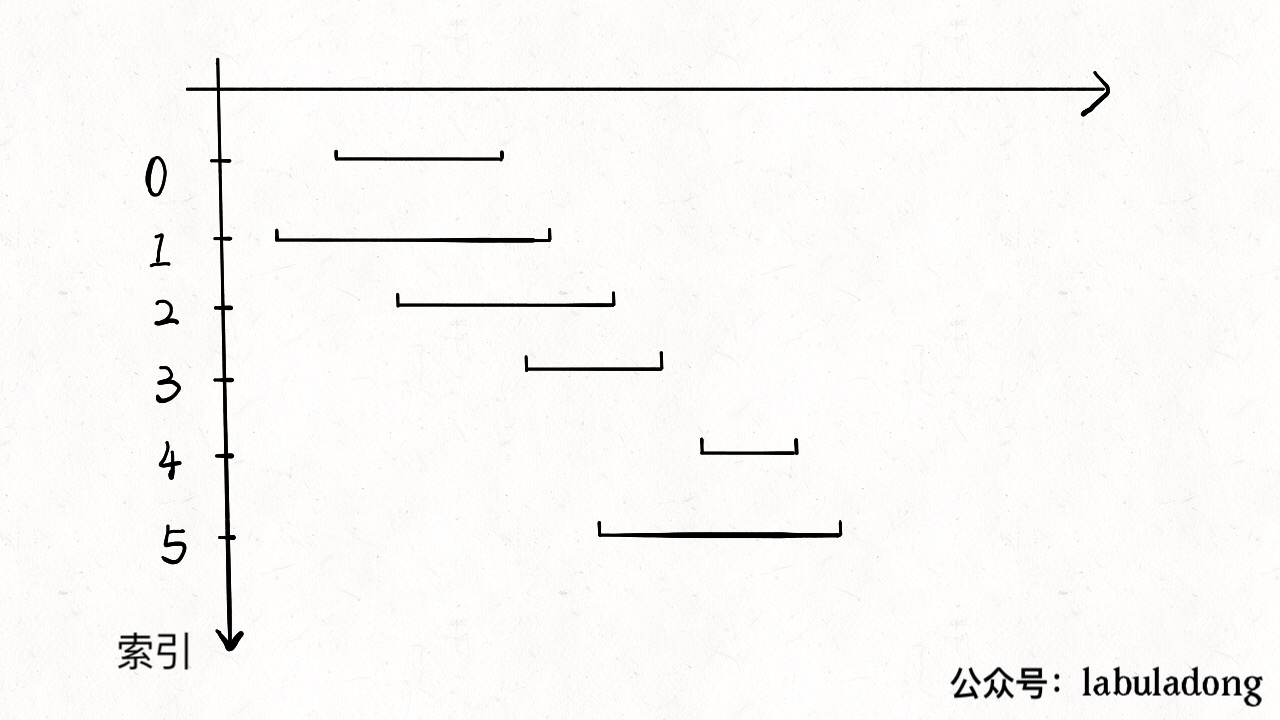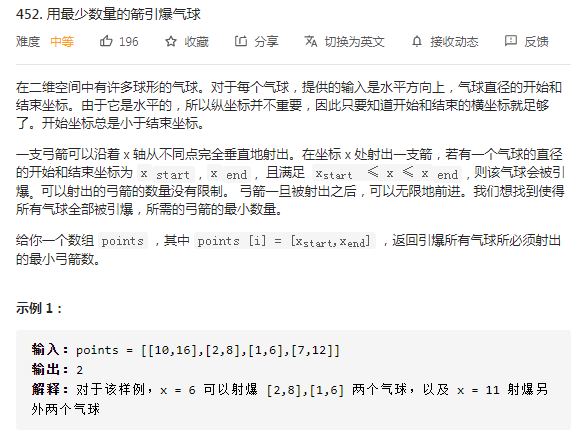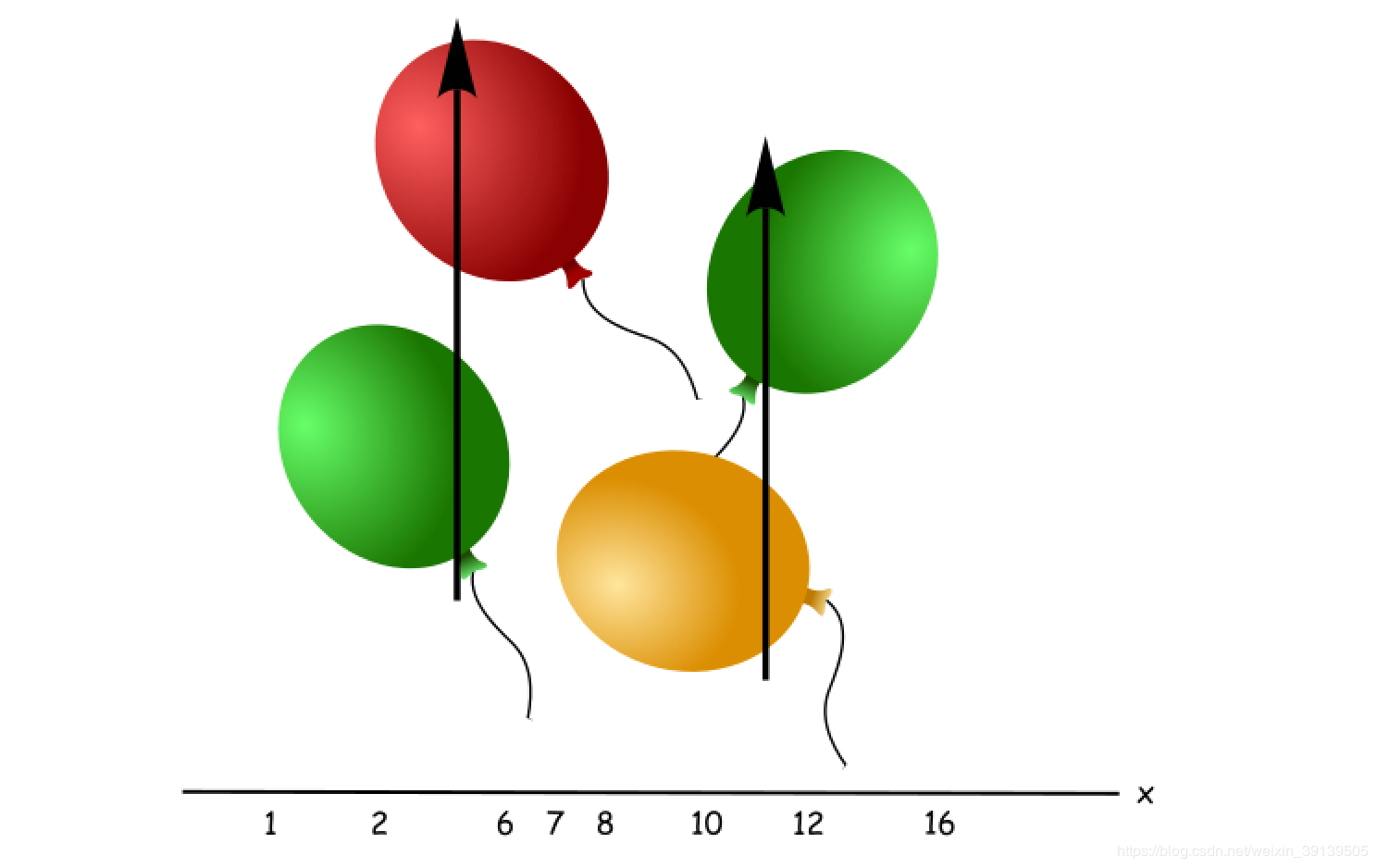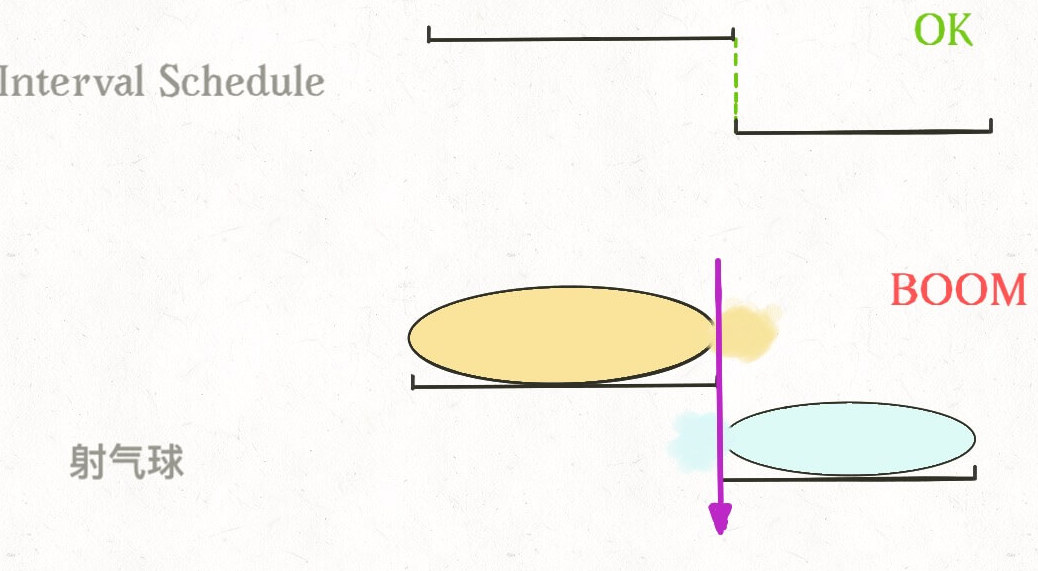# vcjmhg 的个人博客

## 贪心算法原理及其应用# 贪心算法

## 区间调度问题

1. 从区间集合中选择一个区间 x，这个 x 是所有区间中结束最早的（end 最小）。
2. 把所有与 x 区间相交的区间从区间集合中删除掉。
3. 重复 1 和 2，直到区间集合为空。之前选出的那些 x 的集合就是最大的不想交子集。`````` 1 public int eraseOverlapIntervals(int[][] intervals) {
2    if (intervals.length == 0) {
3      return 0;
4    }
5    Arrays.sort(
6        intervals,
7        new Comparator<int[]>() {
8          @Override
9          public int compare(int[] o1, int[] o2) {
10            return o1 - o2;
11          }
12        });
13	//排序后的第一个必然可用
14    int count = 1;
15    int x_end = intervals;
16    for (int[] interval : intervals) {
17      if (interval >= x_end) {
18        count++;
19        x_end = interval;
20      }
21    }
22    return count;
23  }
``````

# 应用`````` 1public int findMinArrowShots(int[][] points) {
2    if (points.length <= 0) {
3      return 0;
4    }
5    // 在排序的过程中要考虑溢出情况的发生
6    Arrays.sort(points, (a, b) -> Integer.compare(a, b));
7    int count = 1;
8    int x_end = points;
9    for (int[] point : points) {
10      if (point > x_end) {
11        count++;
12        x_end = point;
13      }
14    }
15    return count;
16  }
``````

# 总结

1. 在使用考虑贪心算法之前，先考虑使用动态规划（考虑状态压缩）解决该问题，如果问题依然超时，则考虑使用贪心算法。
2. 在确定贪心策略之前，先用一些特殊的例子验证贪心策略的正确性。对于正确的贪心策略，为了保证算法的绝对正确，要通过数学归纳法进行验证。